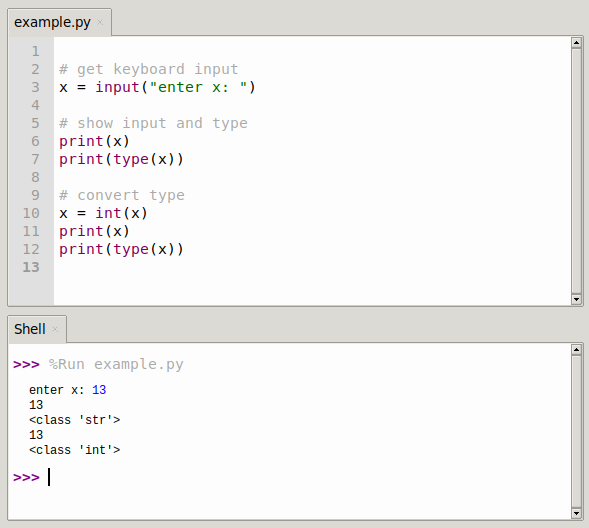# Python input() Function

The input() function takes keyboard input from the console. The input() function accepts a standard input data and returns a string type.

Note: If you stumble upon code in the acient Python2.x, it had the method raw_input(). That doesn’t exist anymore, so use `input()` at all times.

## Python input() example.

### get string from terminal

The input function returns a string by default, so by calling it you get a text object.
Anything returned is of string type (type `str`).

``````>>> name = input("Your name: ")
>>> type(name)
<class 'str'>
>>> print(name)
Shanon
>>> ``````

### get integer from console

The input() function returns a string, not any other data type. That means that if you want to read an integer from the console, you have to cast it:

``````>>> a = input("i: ")
i: 5
>>> a
'5'
>>> type(a)
<class 'str'>
>>> ``````

Type `str`, so if you want an integer, you can do this:

``````>>> a = int(input("i: "))
i: 146
>>> type(a)
<class 'int'>``````### python input list

You can input a list at once, using the code below. The way this works is by getting string input, then splitting the string based on a character like a comma:

``````>>> x = input()
1,2,3,4,5,6

# convert input string into list of strings
>>> xlist = x.split(",")

# output list
>>> xlist
['1', '2', '3', '4', '5', '6']
>>> ``````

But everything is over character value (string), you can see that on the quotes. To convert it to int values, you can do this:

``````>>> xlist = [int(xlist[i]) for i in range(len(xlist))] #for loop, convert each character to int value
>>> xlist
[1, 2, 3, 4, 5, 6]
>>> ``````

The parameters of the `split()` function can be any delimiter, including `(a,b,c...)`; `1,2,3...` ;`1,2,3...` ;`%`,`!` ,`*`, `space`)

``````>>> x=input()
1 2 3 4

# split into list of strings
>>> xlist=x.split(" ")

# show what we have
>>> print(xlist)
['1', '2', '3', '4']

# convert to numbers
>>> xlist = [int(xlist[i]) for i in range(len(xlist))]
>>> print(xlist)
[1, 2, 3, 4]``````